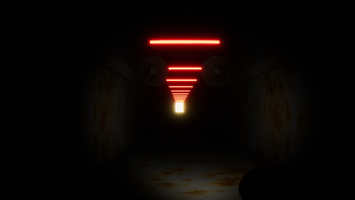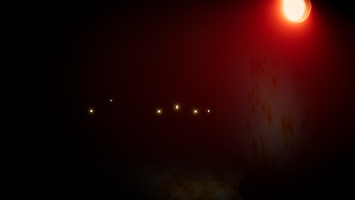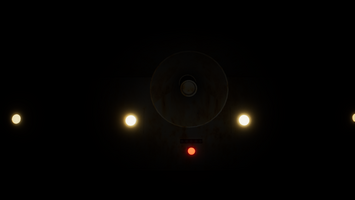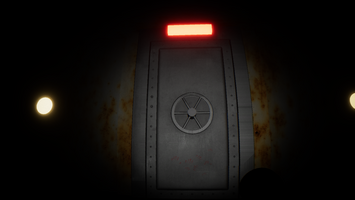# Maze Generation, Pathfinding, and Procedural Animation in "A Way Out"Overview: There are three core technologies which run the systems present in A Way Out. The first is the procedural maze generation. The second is the A* pathfinding algorithm. The third is the procedural animation system which controls the enemies.

Procedural Maze Generation:

The procedural maze generation algorithm present in A Way Out consists of three steps. Before describing the steps for generation we must understand the classes used in the system. The maze is separated into sections comprising four vertices surrounding a central point. Then the sections are placed adjacent to each other from the maze. The walls connecting the vertices of each section are composed of two integers describing the connection between the vertices. For instance [0, 1] describes a line or “maze wall” connecting the bottom left (0) and bottom right (1) vertices.

Step 1 - Loop through each position of the maze. The positions are separated by the size of each section. So if a sectional wall is 6 units long, each section is separated by 6 units on the X axis and 6 units on the Z axis. After each section is created we randomly construct the connections between each vertex of each section, not allowing diagonal connections. After generating the data for each wall we have to create them as Unity GameObjects. However we cannot simply place the walls from one vertex to another since there is overlap between the left vertices of one section and right vertices of its adjacent section (from top vertices of one to the bottom vertices of its adjacent ect...). Therefore when we create the walls they will overlap each other at the corners of each section. This causes severe lighting issues which look absolutely unacceptable. We need to shrink some of the walls so that there is part of a wall present at each corner of each section, but so that no walls overlap. This is covered in Step 2.

Step 2 - There is one easy way to solve this problem. Simply shrink every wall so that no walls appear at the corners of each section. Then fill in each corner with a separate square wall to fill in the gaps. This solution works well, but causes problems when it comes to texturing the walls. Each wall will have a texture applied across it, and each wall will look identical in the texturing as they are all the same scale. But when applying the texture to the smaller square pieces filling in the corners of the maze, the texture will appear squished and look out of place. Therefore we need a different solution. To solve the problem we must return to the walls which would overlap at the corners, scale each wall so that if a wall already appears at a corner, no other wall will overlap it. In order to detect whether or not a wall appears in the corner we can cast a physics ray to where we believe the wall would be (should one be there) and if the ray hits a wall, we know a wall is present. Then we can scale back the wall we are currently placing to account for this. However, an issue arises because when creating a new object in Unity, it is not physically present until the frame after it was created. We must wait a frame before casting a ray to check whether or not a wall is present. To do this we set up a Coroutine instead of a normal function which waits a frame after every wall is placed. This causes the maze to be incrementally generated at about 80 walls per second on average (since the game runs at around 80 frames per second on my fairly average PC). This may seem worse than having the maze generate all at once during one frame, which would be faster overall, but there are advantages to having the game able to run while the maze is being generated. Instead of having to wait around 20 seconds on a loading screen while the maze is being created (it is a rather large maze), we can let the maze generate while the player is doing the tutorial. This effectively makes the loading time for the game almost zero seconds. Sadly however the walls are still being stretched slightly to avoid intersection at the corners, but I assumed it would be small enough so that the textures would not look too “off”. However, this was not the case and the textures still looked strange. Luckily, I had the idea to write a shader that maps the UV coordinates of the texture to the world position of each pixel of each wall so that the texture remained consistent throughout the maze.

Step 3 - The maze is very dense at this point and most likely not possible to complete (complete in this sense means reaching the north side of the maze from the south side). To solve this we randomly delete walls of each section based on the amount of walls of the section. This effectively removes most dead ends, and when tweaked enough, makes the odds of an unsolvable maze astronomical while keeping the tight claustrophobic feeling intact.

A* Pathfinding -

A* is a pathfinding algorithm which A Way Out uses to control the navigation of the monsters in the maze. To use the algorithm we only need a map of nodes which can be transversable or not (blocked by a wall or object). After the maze generation is complete we create a node grid that fills the maze and check whether each node is obstructed by a wall. Each “node” in the grid is a class containing the position of the node in world space, a boolean describing its obstruction state, an empty Node called parent, and finally a G, H, and F, cost value. The F cost is simply the sum of the G and H cost values. The H cost is the distance from the node’s position to the target position and the G cost is the cost of moving to an adjacent node.

The Algorithm -

In short the algorithm progressively determines the shortest possible path from one point to another. We will have two dynamic lists of Nodes which will handle our searching for the shortest path. One list is the open list consisting of nodes which we will search through and a closed list of nodes we have already analyzed. The open list starts consisting of only the node with the closest to the starting position of the path.

1. First find the node in open list with the smallest F-Cost - Set that node as the current node
2. Remove the current node from the open list and add it to the closed list.
3. Check to see if the current is the target node
4. Find all nodes neighboring the current node (not diagonally adjacent!)
5. Foreach of the neighboring nodes do the following
1. If the neighboring node is in the closed list or is obstructed. Skip the node
2. Set the G cost of the neighboring node to the F Cost of the current node
3. Set the H cost (dist from node to target)
4. Set the parent of the neighboring node as the current node
5. If the neighbor node is not in the open list. Add it to the open list
6. Repeat steps 1 - 5 until the open list is emptied or a specified number of loops occur such that the program lags (only to avoid lag).
7. Now we need to extract the final path - Make the current node the target node (as it should be already if you reached this step) and add it to a dynamic list. Then get its parent node and add it to the dynamic list, then get that node's parent and so forth until there is a node without a parent. That will be the starting node. Now reverse that dynamic list. You will have a path of positions that lead to your target.
8. Now move whatever object you want along that path and the pathfinding is done.

To break it down, this algorithm is essentially a breadth first search algorithm which can effectively and efficiently find a path through any valid node map. This algorithm can be very highly optimized and run extremely fast by also implementing techniques from here https://lucho1.github.io/JumpPointSearch/. This project did not require these techniques.

Procedural Animation -

Procedural Animation is an animation technique which, in my case, uses Inverse Kinematics to move joints and limbs to create any number of believable adaptive animations. I used a far more complex procedural animation system in one of my previous projects called “It Sees You” to create a spider which can adapt to any sort of terrain with its movement. This project did not require a procedural animation system since the floor is flat, but I am not able to animate almost anything in a convincing manner, so procedural animation is just an easier solution.

Inverse Kinematics -

Inverse kinematics is a system which finds the angle a series of joints must be at in order to have a node (child of the joints eg. the hip and knee are the joints and the foot is the node) at a certain position. To do this, I used gradient descent due to its simplicity. Gradient descent, essentially, is a system which evaluates one of two options repeatedly in order to find an optimal solution. In the case of trying to move the foot of my creature to a specific position, I rotate the joint along each axis in the positive and negative direction. Whichever direction gets the foot closer to the goal is selected. This repeats until the node is within some threshold of the target. There are more complexities and intricacies involved in the control of these legs, especially so in “It Sees You.” There is not a lot of interesting information in this algorithm that does not involve a deep analysis of this system, so I will leave this here.